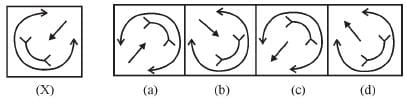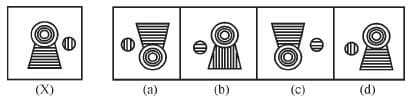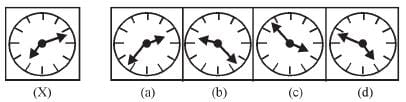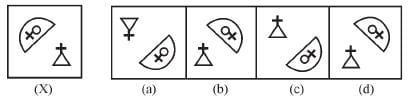Olympiad Test: Mirror Images -1

# Olympiad Test: Mirror Images -1

Test Description

## 20 Questions MCQ Test Science Olympiad Class 6 | Olympiad Test: Mirror Images -1

Olympiad Test: Mirror Images -1 for Class 6 2022 is part of Science Olympiad Class 6 preparation. The Olympiad Test: Mirror Images -1 questions and answers have been prepared according to the Class 6 exam syllabus.The Olympiad Test: Mirror Images -1 MCQs are made for Class 6 2022 Exam. Find important definitions, questions, notes, meanings, examples, exercises, MCQs and online tests for Olympiad Test: Mirror Images -1 below.
Solutions of Olympiad Test: Mirror Images -1 questions in English are available as part of our Science Olympiad Class 6 for Class 6 & Olympiad Test: Mirror Images -1 solutions in Hindi for Science Olympiad Class 6 course. Download more important topics, notes, lectures and mock test series for Class 6 Exam by signing up for free. Attempt Olympiad Test: Mirror Images -1 | 20 questions in 40 minutes | Mock test for Class 6 preparation | Free important questions MCQ to study Science Olympiad Class 6 for Class 6 Exam | Download free PDF with solutions
 1 Crore+ students have signed up on EduRev. Have you?
Olympiad Test: Mirror Images -1 - Question 1

### Directions: In each of the following questions, there is combination of alphabet or number followed by four alternatives. Choose the alternative which most clearly resembles the mirror image of the given combination. TERMINATE

Olympiad Test: Mirror Images -1 - Question 2

### Directions: In each of the following questions, there is combination of alphabet or number followed by four alternatives. Choose the alternative which most clearly resembles the mirror image of the given combination. 1965INDOPAK

Olympiad Test: Mirror Images -1 - Question 3

### Directions: In each of the following questions, there is combination of alphabet or number followed by four alternatives. Choose the alternative which most clearly resembles the mirror image of the given combination. NATIONAL

Olympiad Test: Mirror Images -1 - Question 4

Directions: In each of the following questions, there is combination of alphabet or number followed by four alternatives. Choose the alternative which most clearly resembles the mirror image of the given combination.

UTZFY6KH

Olympiad Test: Mirror Images -1 - Question 5

Directions: In each of the following questions, there is combination of alphabet or number followed by four alternatives. Choose the alternative which most clearly resembles the mirror image of the given combination.

SUPERVISOR

Olympiad Test: Mirror Images -1 - Question 6

Directions: In each of the following questions, there is combination of alphabet or number followed by four alternatives. Choose the alternative which most clearly resembles the mirror image of the given combination.

JUDGEMENT

Olympiad Test: Mirror Images -1 - Question 7

Directions: In each of the following questions, there is combination of alphabet or number followed by four alternatives. Choose the alternative which most clearly resembles the mirror image of the given combination.

ANS43Q12

Olympiad Test: Mirror Images -1 - Question 8

Directions: In each of the following questions, there is combination of alphabet or number followed by four alternatives. Choose the alternative which most clearly resembles the mirror image of the given combination.

BRISK

Olympiad Test: Mirror Images -1 - Question 9

Directions: In each of the following questions, there is combination of alphabet or number followed by four alternatives. Choose the alternative which most clearly resembles the mirror image of the given combination.

EFFECTIVE

Olympiad Test: Mirror Images -1 - Question 10

Directions: In each of the following questions, there is combination of alphabet or number followed by four alternatives. Choose the alternative which most clearly resembles the mirror image of the given combination.

TARAIN1014A

Olympiad Test: Mirror Images -1 - Question 11

Directions: In each of the following questions, there is combination of alphabet or number followed by four alternatives. Choose the alternative which most clearly resembles the mirror image of the given combination.

MAGAZINE

Olympiad Test: Mirror Images -1 - Question 12

Directions: In each of the following questions, there is combination of alphabet or number followed by four alternatives. Choose the alternative which most clearly resembles the mirror image of the given combination.

BR4AQ16HI

Olympiad Test: Mirror Images -1 - Question 13

Directions: In each of the following questions, there is combination of alphabet or number followed by four alternatives. Choose the alternative which most clearly resembles the mirror image of the given combination.

DL9CG4728

Olympiad Test: Mirror Images -1 - Question 14

Directions: In each of the following questions, there is combination of alphabet or number followed by four alternatives. Choose the alternative which most clearly resembles the mirror image of the given combination.

IMFORMATIONS

Olympiad Test: Mirror Images -1 - Question 15

Directions: In each of the following questions, there is combination of alphabet or number followed by four alternatives. Choose the alternative which most clearly resembles the mirror image of the given combination.

REASONING

Olympiad Test: Mirror Images -1 - Question 16

Directions: In each of the following questions, there is combination of alphabet or number followed by four alternatives. Choose the alternative which most clearly resembles the mirror image of the given combination.

AN54WMG3

Olympiad Test: Mirror Images -1 - Question 17

Directions: In each of the following questions, choose the correct mirror image of the figure (X) from amongst the four alternatives (a), (b), (c) and (d) given along with Fig. (X).Olympiad Test: Mirror Images -1 - Question 18

Directions: In each of the following questions, choose the correct mirror image of the figure (X) from amongst the four alternatives (a), (b), (c) and (d) given along with Fig. (X).Olympiad Test: Mirror Images -1 - Question 19

Directions: In each of the following questions, choose the correct mirror image of the figure (X) from amongst the four alternatives (a), (b), (c) and (d) given along with Fig. (X).Olympiad Test: Mirror Images -1 - Question 20

Directions: In each of the following questions, choose the correct mirror image of the figure (X) from amongst the four alternatives (a), (b), (c) and (d) given along with Fig. (X).## Science Olympiad Class 6

16 videos|42 tests
 Use Code STAYHOME200 and get INR 200 additional OFF Use Coupon Code
Information about Olympiad Test: Mirror Images -1 Page
In this test you can find the Exam questions for Olympiad Test: Mirror Images -1 solved & explained in the simplest way possible. Besides giving Questions and answers for Olympiad Test: Mirror Images -1, EduRev gives you an ample number of Online tests for practice

## Science Olympiad Class 6

16 videos|42 tests